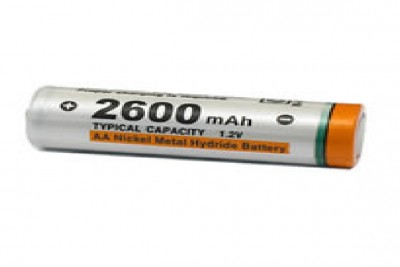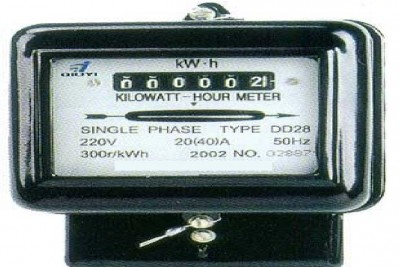# Difference between MAH and WH

Hand held or portable devices are getting very popular and common nowadays. The reason for this is that these devices use powered batteries that are made in such a way that they can utilize least amount of energy. Since the amount of energy they pull is small therefore small units are required to represent it. Two units in this regard are MAH and WH.

The difference between mAh (milli Ampere hour) and Wh (Watt hour) is that mAh a unit of charge capacity or storage while Wh (Watt hour) is a unit of energy storage and amount.

### Instructions

• 1

MAH (milli Ampere hour):

Milli Ampere hour (mAh) is defined as one thousandth of an Ampere hour which is is a unit of electric charge. Ampere hour is used to represent an important fact that if an electric device utilizes or gives 1 Ampere of current in an one hour without any delays, then 3600 Coulombs of charge is passed. Therefore this implies that one milli-Ampere hour is equal to 3.6 Coulombs.

Here the concept of current is very important and is defined as:

Current ( I ) = (charge flow)/time = ΔQ/Δt

The above equation can also be written like this: ΔQ=I×Δt.

This equation states that if we multiply current and time then it results in the value of charge passed within that time duration Δt.

This unit is used to represent energy in the electro-chemical systems. In addition to this, mAh is also used to represent the value of energy in the batteries of laptops.

- Image Courtesy: differencebetween.info• 2

WH (Watt hour):

Watt hour measures the energy.  For Watt hour if the amount of energy consumed or generated by an electric device is operated continuously with a power of 1 watt for 1 hour then it is equal to 3600 joules.

The main energy power units are always recorded using Wh unit. Power station outputs are often given in megawatt hours (MWh) while domestic electricity is recorded in kilo watt hours (kWh). [1kWh = 1000Wh = 3.6 MJ (mega joules) and 1 MWh = 1000000 Wh = 3.6 GJ (Giga joules)]

Consider the definition of power:

Power ( P ) = (Energy Used)/time = ΔE/Δt

The above equation can also be written like this: ΔE=P× Δt . This states that the product of the power and time gives the energy which is generated during the time interval Δt.

Batteries give relatively lower power and hence watt hour (Wh) is used as a unit in batteries.•